Name:    Chapter 3 Test

Multiple Choice
Identify the choice that best completes the statement or answers the question.

Simplify the expression.

1.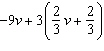a. –7v – 2 c. 7v + 2 b. –7v + 2 d. –7v +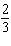2.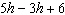a.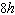c.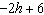b.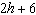d.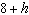3.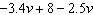a.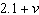c.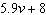b.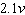d.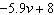4.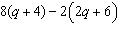a.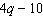c.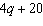b.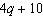d.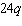Find the difference.

5.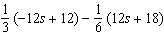a.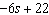c.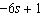b.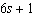d.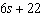6.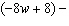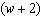a.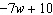c.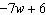b.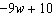d.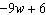Find the sum.

7.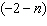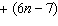a.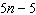c.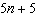b.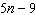d.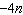8.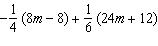a.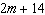c.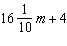b.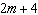d.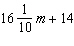Factor out the coefficient of the variable.

9.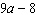a.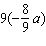c.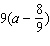b.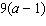d.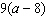10.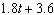a.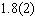c.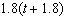b.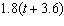d.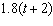Solve the equation. Check your solution.

11.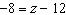a. 0.67 c. 4 b. –4 d. –20

12.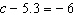a. –1.13 c. –0.7 b. –11.3 d. 11.3

13.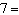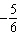c
 a.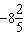c.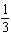b.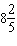d.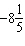14.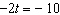a. 20 c. –12 b. –8 d. 5

15.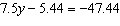a. –5.6 c. –54.94 b. –42 d. –52.88

16.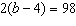a. 53 c. 49 b. 106 d. 212

Write an equation. Then solve.

1.

The difference between the lengths of a paddle boat and a pier is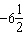feet. The pier is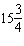feet long. How long is the paddle boat?

2.

A rock climber averages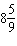feet per minute. How many feet does the rock climber climb in 50 minutes?

3.

You are riding a personal watercraft at a steady cruising speed toward a dock 3050 feet away. You travel at this speed for 55 seconds. You begin to slow down 300 feet from the dock. Write and solve an equation to find your cruising speed s in feet per second.

4.

You and a friend decide to sell cookies to raise money for a local fundraiser. After d days, you have raised (9d + 10) dollars and your friend has raised (8d + 5) dollars. Write an expression that represents the total amount of money that you raised together.